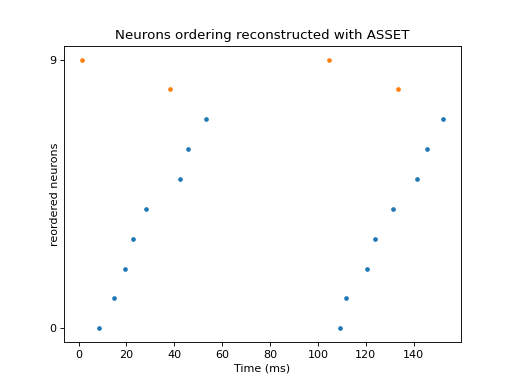# viziphant.asset.plot_synchronous_events¶

viziphant.asset.plot_synchronous_events(spiketrains, sse, title=None, **kwargs)[source]

Reorder and plot the spiketrains according to a series of synchronous events sse obtained with the ASSET analysis. Spike trains that do not participate in the chosen group will be shown at the top in a different color.

Parameters
spiketrainslist of neo.SpikeTrain

ASSET input spiketrains.

ssedict

One entry of the output dict from `elephant.asset.ASSET.extract_synchronous_events()`.

titlestr or None, optional

User-defined title string. If None, it’ll be set to an automatic description. Default: None

**kwargs

Additional arguments to `viziphant.rasterplot.rasterplot()`

Returns
axesmatplotlib.Axes.axes

`viziphant.patterns.plot_patterns`

plot patterns repeated in time

Examples

In this example we

• simulate two noisy synfire chains;

• shuffle the neurons to destroy visual appearance;

• run ASSET analysis to recover the original neurons arrangement.

```import neo
import numpy as np
import quantities as pq
import matplotlib.pyplot as plt

import viziphant
from elephant import asset

np.random.seed(10)
spiketrain = np.linspace(0, 50, num=10)
np.random.shuffle(spiketrain)
spiketrains = np.c_[spiketrain, spiketrain + 100]
spiketrains += np.random.random_sample(spiketrains.shape) * 5
spiketrains = [neo.SpikeTrain(st, units='ms', t_stop=1 * pq.s)
for st in spiketrains]
asset_obj = asset.ASSET(spiketrains, bin_size=3 * pq.ms)

imat = asset_obj.intersection_matrix()
pmat = asset_obj.probability_matrix_analytical(imat,
kernel_width=50 * pq.ms)
jmat = asset_obj.joint_probability_matrix(pmat, filter_shape=(5, 1),
n_largest=3)